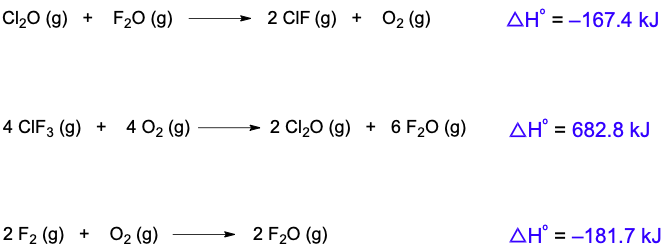## General Chemistry

Learn the toughest concepts covered in Chemistry with step-by-step video tutorials and practice problems by world-class tutors

8. Thermochemistry

# Hess's Law

Hess's Law involves the use of partial reactions in the determination of the overall enthalpy of reaction.

Hess's Law
1
concept

## Hess's Law3m
Play a video:
Now, Hess's law has to do with the rearrangement of thermo chemical equations to help us find the overall entropy of reaction. Now recall. A thermal chemical equation is a chemical equation that involves or includes an entropy of reaction. Now we're going to say the thermal chemical equation, and it's NLP of reaction, which is Delta h r x n are directly proportional. Okay, this means that any change to the original equation will cause the same change within your entropy of reaction. So let's say we have an original equation here. Original thermal chemical equation where we have two moles of magnesium, a solid plus o two gas, and it produces two moles of magnesium oxide. The entropy associated with this is negative. 12 04 killer jewels. Now I can do different things different rearrangements to this thermal chemical equation that will affect it but also affect my delta h of reaction. So the things I can do is I can multiply this equation by a value I can divide it by a number, or I can reverse the reaction. So let's say we multiply it here we have our original equation and let's say multiply it by two. Now, if I multiply it by two, the coefficients not become 42 and four. If I multiply the equation by two, then that means I also have to multiply my delta H by two. So this original value of negative 12 04 killer Jules gets multiplied by two. Okay? And now my new entropy of the reaction is negative. 24 08 killer jewels. Now, let's say instead of multiplying, I divided. So my original equation again. Now I, divided by two, dividing it by two changes my coefficients now to one toe, one for magnesium, solid to one half for and one for magnesium oxide. Again, Whatever I do to the equation, I must do the same thing to my delta h So negative 12 04 gets divided by two. So it becomes negative. 602 killer jewels. Is it? Finally, the last thing I could do to my thermo chemical equation? As I can reverse it, what was a product can become a reactant. So that's two m g o solid. And what was a reactant cannot become products. So two mg solid + Now, when I reverse the reaction. What that does is it reverses the sign of Delta H. So it was a negative 12. 04 Now it's gonna become a positive 12. 04 So just realize that if I reverse the reaction, I reverse the sign of Delta H. So just remember, these are the three things I could do toe a thermal chemical equation, and they have a direct impact on my delta h of reaction for that particular thermal chemical equation.

Multiplication of the original reaction results in multiplication of ∆Hrxn.

Division results in the division of ∆Hrxn..

Reversing the original reaction results in reversing the sign of ∆Hrxn.

2
example

## Hess's Law Example 11m
Play a video:
in this example question. It says, if the formation equation for boron trioxide is given as the following four moles of Be solid plus three moles of +02 gas produces two moles off boron trioxide Solid has an entropy of negative 25 47 killing jewels. What will be the new entropy value when it's rearranged? All right, so we have to see what the changes that the original equation under wind to determine the new Delta h Well, we can see one thing that happened is what was a product is now a reactant and what was a reactant are now products. So we reversed it. Yeah, the next thing we should notice are the coefficients here it was a two, but now it's a four. This was a four, but now it's an eight. This was a three banal to six, so we didn't Onley reverse the reaction, but we multiplied by two. Yeah, Now, remember, when you reverse the reaction that's going to reverse the sign of Delta H. So now it's gonna be positive and whatever number I multiply my equation by, I multiply Delta H by that same number. So you multiply this by two. When you do that, it gives you 50 94 killer jewels. So for my new equation would be a positive 5000 and killer jewels. Reversing the reaction reverses the sign. Multiplying it by a value means I multiply my delta h value by that same number.
3
Problem

Calculate the ∆Hrxn for the following thermochemical equation: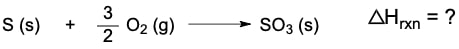When given the following: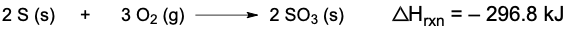4
concept

## Hess's Law2m
Play a video:
many reactions cannot be carried out in a single step, but instead require multiple steps to get to the final product. We're gonna say Hess's law states that the entropy of reaction often overall reaction is the sum of the entropy is the standard. Entropy values off these multiple steps. So here we have a partial reaction one and a partial reaction to By combining them together, they helped to give me my overall reaction down here. Now what we do is this Zenon die Fluoride is reacting so it comes down. Then we're gonna say here that this flooring, which is a solid I'm not not solid. A product cancels out with one of these florins, which is a reactant, remember, they can't exist on both sides. OK, this causes an imbalance. These air called reaction intermediates and they will cancel one another out. So this cancels out with one of these leaving us with one behind this Zen in here is a product. And this one here is a reactant again can exist is both. There's one and one on each, so they both cancel out each other entirely. What's left behind comes down. So this f two comes down to give me this reactant, and then this Zenon try. Florida comes down to give me this final product to find the overall entropy of reaction. For this overall reaction, you add up each of these partial standard entropy values, So when you add 1 23 and you subtracted, subtract 2 62. That's how we come up with this new Delta h of reaction. That's a negative 1. 39 Kill a Jules. This, in essence, is what Hess's law tries to do. It takes partial reactions and from them helps us to determine the overall reaction and associated with it in overall entropy of reaction.

Addition of ∆Hrxn values of multiple steps gives us the ∆Hrxn of the overall reaction.

5
example

## Hess's Law Example 25m
Play a video:
here, it says to calculate the MPP of reaction for the overall reaction. So we have carbon monoxide plus nitrogen monoxide producing carbon dioxide and half a mole of nitrogen gas. Now here were given these partial reactions below with their own known standard mantelpiece off reaction. Now what do we do? Well, let's follow the rules. Step one says that I need to start with the first compound in the overall equation and located in the set of partial reactions. Compound from partial reaction must match in terms of number and type. We'll see what that means with one from the overall equation. This may require you to reverse, multiply or divide the partial reaction, which will also affect your standard entropy value. Step two says. Keep moving on to the next compound in the overall equation until locate all compounds in the partial reaction. So let's do steps one and two initially, all right, so we have to. So if we go back to step one, it says that we need to locate the compound. We must locate the first comment in the overall equation and located in the set off partial reactions. Alright, so in my overall equation. I have carbon monoxide gas as a reactant, and there's one of it, one mole of it. So if I look in the partial reactions, we see carbon monoxide is right here. But we have an issue. Although there is one mole of it like I want it is a product we needed to match up with the overall equation. We needed to be a reactant to do that. That means I'm gonna have to reverse the reaction. Now when I reverse the reaction, it's now gonna become our reactant. Oh, to also becomes a reactant and co two becomes a product. Now, remember, when I reverse the reaction, that's going to reverse the sign for Delta H. So now, my carbon monoxide, there's one mole of it. So the number is good and the type is also good because it's also reacted now, just like in my overall equation. This equation is gone now because it's been reversed and is represented by this bottom one. Next, I need to find no gas. I needed to be one mole of it, and I needed to be a reactant. Here I see it. Two issues First, it has a two in front of it. I needed to have a coefficient of one, just like in my overall equation. Also, I needed to be a reactant. So what I'm gonna do here is I'm going to divide by two and reverse. Okay, so here, I'm gonna divide by two and reverse it. So that's gonna become an out gas. And then when I reverse and when I divide by two, this becomes half end to okay. Plus half +02 when I reversed the equation. Yeah. Remember that's going to change. The science of this becomes negative. And when I divide by two, I have to divide Delta H by two. So this is negative. 90.3 killer jewels. So now this animal matches up exactly with the overall equation. Keep going. We need one mole of co two, his product, which we have here. We'd have a mole of and to as product, which we have here. So all the compounds I need match up with the overall equation. So now we go to Step three, combine the partial reactions and cross that reaction intermediates if present reaction intermediates or compounds that look the same with one as a reactant and the other as a product. Once we do that, we go to step forward. We add all the standard entropy, values off my partial reactions to obtain the entropy of reaction of the overall reaction. All right, so who are intermediates? Things that look the same but one's a product and one's reacted. We have half Oh, to here as a reactant which will cancel out with this half 02 That's a product. Those are Onley reaction intermediates. Everything else comes down. If you look when everything comes down, it should give you back the overall equation you are looking for in the first place. It doesn't matter. The order that you write them in All that matters is that the coefficients match the overall equation and that they're on the right side. Reactant is here. Should match up with the reactions up in the overall products here should match up with the products up here. All we do now is we add up these mental piece of reaction. So when I add up negative to 83 plus this negative 90.3, that gives me a total entropy off reaction equal to negative 3. 73.3 killer jewels. So this would be our Hess's law question where we solved for the overall entropy of the reaction for the overall reaction.
6
Problem

Calculate the ∆Hrxn for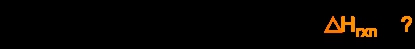Given the following set of reactions: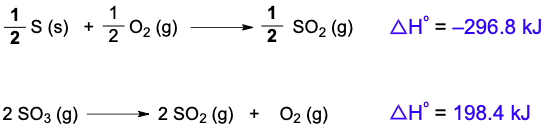7
Problem

Calculate the ∆Hrxn for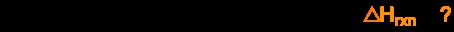Given the following reactions: